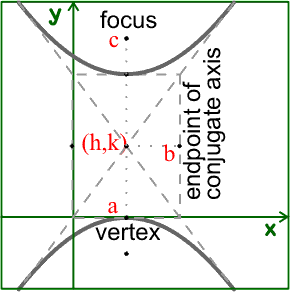• If you are citizen of an European Union member nation, you may not use this service unless you are at least 16 years old.

View

# Hyperbolas

last edited by 11 years, 7 months agoHyperbola- Set of all points the difference of whose distances from 2 fixed points is constant

TRANSLATION:

Looks like two parabolas going in opposite directions. The center is the same distance from each of the two vertices. There is one vertex on each part on the hyperbola, and it is on the part of the curve closest to the center. The hyperboles curve away from the center, vertically or horizontally.

LOOKS LIKE:Both the horizontal and vertical hyperbolas have standard equations to find the center(h,k), the distance between the vertices( transverse axis, a), and the conjugate axis(b) which is an imaginary axis which hints to any asymptotes.

There are two standard equations for hyperbolas:

If horizontal, they cross the x axis:=1If vertical, they cross the y axis:=1There are two points within the hyperbolas that are equidistant from the center, called foci. They are labeled in the graph above as point c.

To find the distance from the center to the foci the equation:is used.

To find the exact points of the foci,

it depends on if the hyperbola is vertical or horizontal!!!!!!!!!

HORIZONTAL

• Lets say you solve the problem above and you find c= 5 and -5.

• If the hyperbola is going horizontally, add 5 and subtract 5 from the x value of the center (h).

• For example if the center was at (2,3) the foci would be at (7,3) and (-3,3)

VERTICAL

• You find c=5 and -5 again.
• If the hyperbola is vertical, add 5 and subtract 5 from the y value of the center (k).
• If the center was at (0,2) the foci would be at (0,7) and (0,-3)For further information visit some of these sites:

If you really want to see a video about how to solve these see this link